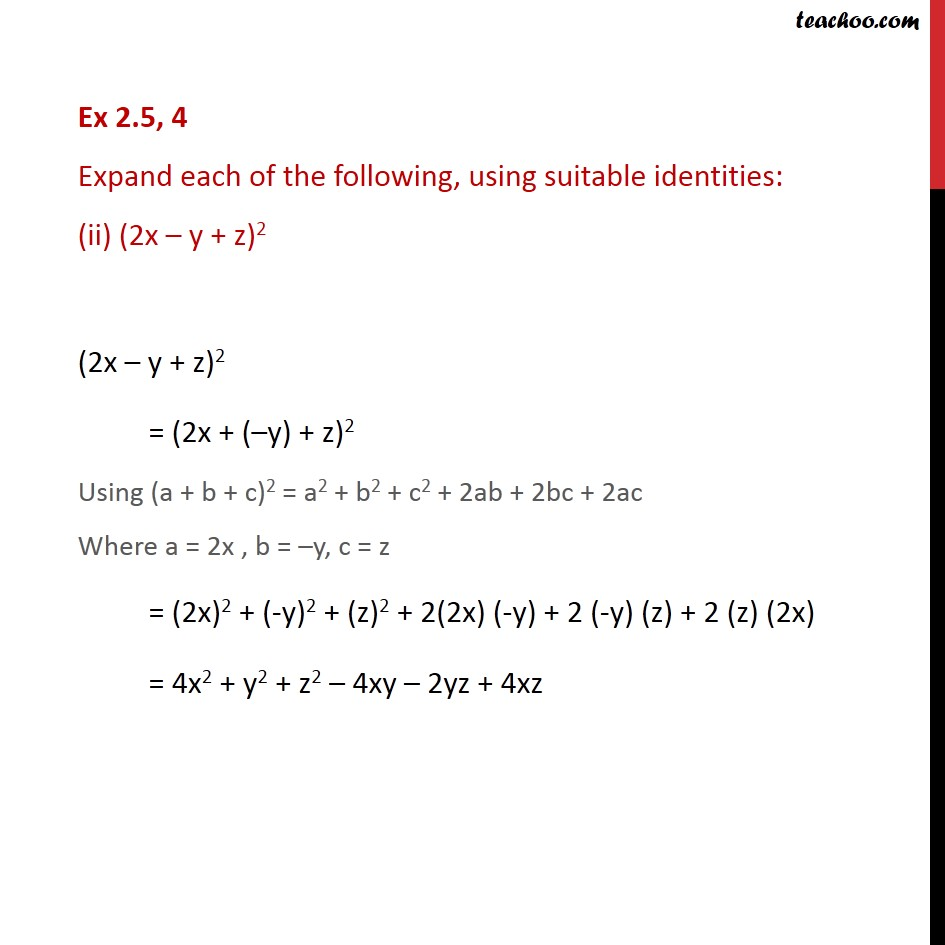Ex 2.4

Chapter 2 Class 9 Polynomials
Serial order wiseLearn in your speed, with individual attention - Teachoo Maths 1-on-1 Class

### Transcript

Ex 2.4, 4 Expand each of the following, using suitable identities: (ii) (2x y + z)2 (2x y + z)2 = (2x + ( y) + z)2 Using (a + b + c)2 = a2 + b2 + c2 + 2ab + 2bc + 2ac Where a = 2x , b = y, c = z = (2x)2 + (-y)2 + (z)2 + 2(2x) (-y) + 2 (-y) (z) + 2 (z) (2x) = 4x2 + y2 + z2 4xy 2yz + 4xz